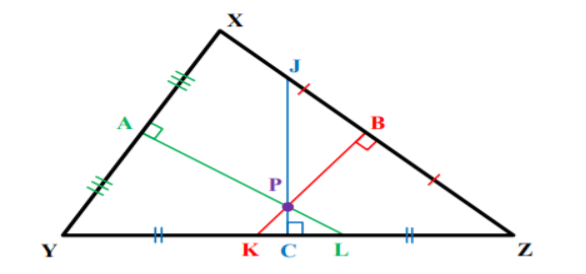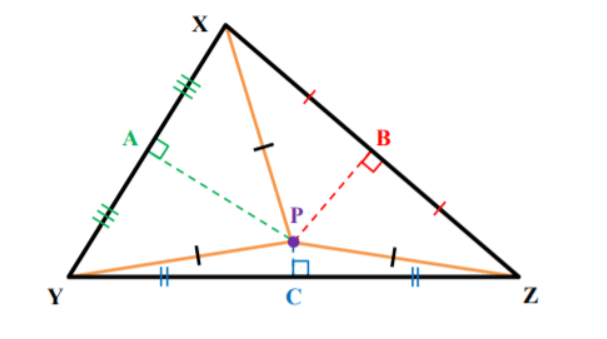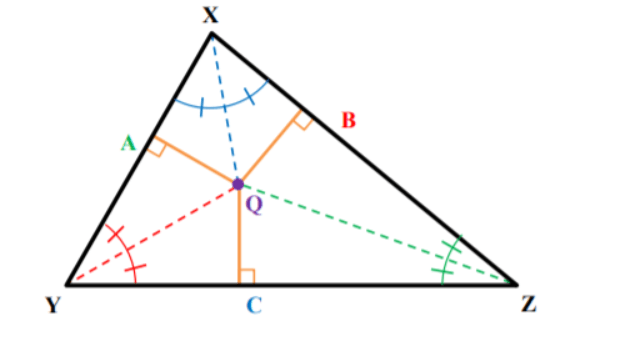# Teaching Bisectors in Triangles

In earlier lessons, students have familiarized themselves with perpendicular and angle bisectors. They’re now ready to learn about bisectors in triangles, and more specifically, how to apply the properties of perpendicular and angle bisectors of a triangle.

Although teaching bisectors in triangles can be challenging, there are some ways to make your lesson more interesting. So if you’re teaching this topic, here are some great guidelines that you can follow to help you best prepare for success in your lesson!## Guidelines for Teaching Bisectors in Triangles

### Review Perpendicular and Angle Bisectors

You can start your lesson by providing a short overview of what students have already learned on bisectors. Remind them that bisectors are the things that bisect an object into two equal parts. This can be a line bisecting angles, or a line bisecting line segments.

Then, remind students that a perpendicular bisector is a line segment, line, a ray, or a plane that is perpendicular to another segment at its midpoint. As an example, we can imagine it as a line intersecting a line segment at 90 degrees and cutting it into two equal parts.

Finally, refresh students’ knowledge of angle bisectors. Point out that an angle bisector is a line, segment, or ray that cuts an angle in two equal parts. Ask students to draw a perpendicular bisector and an angle bisector as bell-work activity.

### Perpendicular Bisectors of a Triangle

Explain to students that when we have segments, rays, or lines that intersect a side of a triangle at 90 degrees at its midpoint, we call them perpendicular bisectors of a triangle. Add that all triangles have three perpendicular bisectors. Illustrate this with a drawing:Explain which are the three perpendicular bisectors of the triangle XYZ in the drawing, that is:

• line AL is a perpendicular bisector of this triangle because it intersects the side XY at an angle of 90 degrees at its midpoint
• line BK is a perpendicular bisector of this triangle because it intersects the side XZ at an angle of 90 degrees at its midpoint
• line JC is a perpendicular bisector of this triangle because it intersects the side YZ at an angle of 90 degrees

### Circumcenter Theorem

Explain that the point where three or more lines, rays, segments intersect is called a point of concurrency. In a triangle with perpendicular bisectors, this point is known as the circumcenter of a triangle, i.e. the point of concurrency of the three perpendicular bisectors of a triangle.

Ask students to observe the above drawing and identify its circumcenter. They should be able to easily spot that the circumcenter of the triangle XYZ is point P.

Then, explain that the circumcenter theorem states that the circumcenter of a triangle is equidistant from the vertices of the triangle. In the drawing below, this means that line PX = line PY = PZ.### Angle Bisectors of a Triangle

Make sure to refresh students’ understanding of vertices. Students should already know that the vertices of a triangle are basically the corners of the triangle. So every triangle has three vertices. Add that the singular form of vertices is vertex.

Explain to students that angle bisectors of a triangle are segments, rays, or lines that intersect a vertex of a triangle, dividing an angle into two congruent adjacent angles. Every triangle has three angle bisectors.### Incenter Theorem

The angle bisectors of a triangle all meet at one single point. This holds true for all types of triangles – acute, obtuse, scalene, isosceles, etc. The point where the three angle bisectors of a triangle meet is called the incenter. Illustrate angle bisectors and the incenter with a drawing:Point out that this triangle has three angle bisectors, including line AZ, line BY, and line CX, all of them dividing the three angles of the triangle into two equal parts. Add that the incenter in this drawing is point Q, representing the point of concurrency of these three lines.

Explain to students that the incenter theorem states that the incenter of a triangle is equidistant from the sides of the triangle, i.e. the distances between this point and the sides are equal. Illustrate the incenter theorem with a drawing on the whiteboard:Explain that based on this drawing, we can also say that line AQ = BQ = CQ. Add that the incenter actually represents the center of a circle. This means that lines AQ = BQ = CQ are equal to the radius of the circle.

This circle is actually the largest circle that can fully fit into a given triangle. Sometimes it is referred to as an incircle. You can also draw a circle inside the triangle to help students visualize this better.

You could also use videos in your lesson. For instance, use this video to introduce students to angle bisectors in a triangle and the point where these bisectors meet. The video uses a lot of practical examples with illustrative drawings, which students are bound to enjoy.

In addition, this video provides a simple explanation of what the incenter and incircle of a triangle are and how to find them using angle bisectors. Finally, this video provides an overview of the circumcenter of a triangle.

## Activities to Practice Bisectors in Triangles

### Pair Work

This is a simple activity that will help students reinforce their knowledge of bisectors in triangles, as well as learn how to apply the properties of perpendicular and angle bisectors of a triangle. To use this activity in your class, you’ll need to print out this Assignment Worksheet (Members Only).

Pair students up and hand out the worksheets. Explain that the worksheet contains several exercises related to bisectors in triangles. Students in each pair work together to solve the exercises. In the end, provide time for discussion and reflection.

## Before You Leave…

If you liked our strategies on teaching bisectors in triangles, and you’re looking for more math resources for kids of all ages, sign up for our emails to receive loads of free resources, including worksheets, guided lesson plans and notes, activities, and much more!

Feel free to also head over to our blog, where you’ll find plenty of free and awesome resources! And if you’d like to become a member, join us in the Math Teacher Coach community!